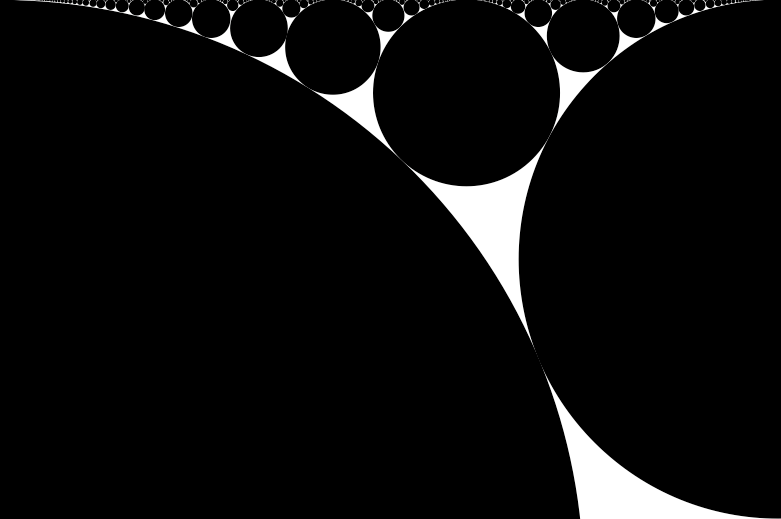## Ford Circles

I picked up a copy of The Math Book after reading the review on John D. Cook's blog.

So far it has been pretty interesting and I've was plesently surprised with how much of the material I am familar with, despite the fact that I haven't studied math beyond the courses that are part of the engineering curriculum at my university.

The best part, though, has been the occasions when I find something that I can play with a little bit on my own. A good example is Ford Circles, which with a little time in NodeBox I had my own renderings:Here's the code:

```from __future__ import division

scale(1000, 1000)

def circle(x, y, r):
oval(x * 1000, y * 1000, 2 * r, 2 * r)

def ford(h, k):
r = 1 / (2 * k**2)
x, y = h/k, r
print x, y, r
circle(x, y, r)

for h in range(0, 200):
for k in range(1, 200):
ford(h, k)
```

... and here's a bigger rendering, if you're interested.